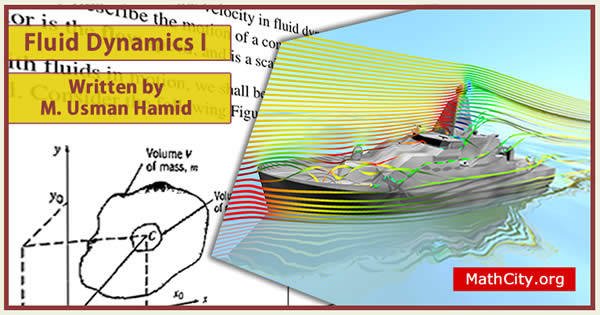# Fluid Dynamics I by Muhammad Usman HamidThese notes are send by Mr. Anwar Khan. We are really very thankful to him for providing these notes and appreciates his effort to publish these notes on MathCity.org. These notes are written by Muhammad Usman Hamid.

 Name Fluid Dynamics I Muhammad Usman Hamid 159 pages PDF (see Software section for PDF Reader) 3.64 mB

Introduction: Definition of Fluid, basics equations, Methods of analysis, dimensions and units. Fundamental concepts, Fluid as a continuum, velocity field, stress field, viscosity, surface tension, description and classification of fluid motions.

Fluid Statics: The basic equation of fluid static, The standard atmosphere, pressure variation in a static fluid, fluid in rigid body motion. Basic equation in integral form for a control volume, basic laws for a system, relation of derivatives to the control volume formulation, conservation of mass, momentum equation for inertial control volume, momentum equation for control volume with rectilinear acceleration, momentum equation for control volume with arbitrary acceleration, the angular momentum principle, the first law of thermodynamics, the second law of thermodynamics.

Introduction to differential analysis of fluid motion: conservation of mass, stream function for two dimensional incompressible flow, motion of a fluid element (kinematics), momentum equation.

Books Recommended:

• Fox R. W. & McDonald A. T. Introduction to Fluid Mechanics.
• White F. M. Fluid Mechanics.
• Schichting H. Boundary Layer Theory.
• Milne-Thomson L. M. Theoretical Hydrodynamics.

• msc/notes/fluid-dynamics-i-m-usman-hamid